TitleCollege Algebra
Answer/Discussion to Practice Problems
Tutorial 58: ProbabilityAnswer/Discussion to 1a Find the probability that a student's favorite type of music is jazz.  Round answer to three decimal places.

 To find this probability, we need to know n(E) = n(students whose favorite type of music is jazz), which is the number of students who preferred jazz and n(S) = n(students surveyed), which is the total number of students surveyed. What do you think n(E) = n(students whose favorite type of music is jazz) is?  If you said 24 you are correct.  24 students indicated that their favorite type of music is jazz.   What do you think n(S) = n(students surveyed) is?  If you said 128 give yourself a pat on the back.  If you total 51 + 24 + 33 + 5 + 15 you get 128. Putting this together in the Empirical Probability formula you get: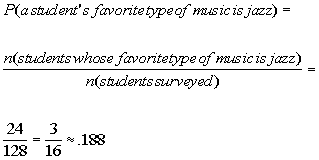*putting in the numbers found above *reducing the fraction  *rounding to 3 decimal places

 The probability that a student's favorite type of music is jazz is .188.Answer/Discussion to 1b Find the probability that a student's favorite type of music is classical.   Round answer to three decimal places.

 To find this probability, we need to know n(E) = n(students whose favorite type of music is classical), which is the number of students who preferred classical and n(S) = n(students surveyed), which is the total number of students surveyed. What do you think n(E) = n(students whose favorite type of music is classical) is?  If you said 5 you are correct.  5 students indicated that their favorite type of music is classical.   What do you think n(S) = n(students surveyed) is?  If you said 128 give yourself a pat on the back.  If you total 51 + 24 + 33 + 5 + 15 you get 128. Putting this together in the Empirical Probability formula you get: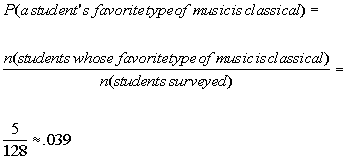*putting in the numbers found above *rounding to 3 decimal places

 The probability that a student's favorite type of music is classical is .039.Answer/Discussion to 2a Find the probability that you are dealt a jack.  Round answer to three decimal places.

 To find this probability we need to know n(E) = n(jacks), which is the number of jacks in a deck of cards and n(S) = n(cards in a deck), which is the total number of cards in a deck. What do you think n(E) = n(jacks) is?  If you said 4 you are correct.  There are 4 jacks in a deck of cards.   What do you think n(S) = n(cards in a deck) is?  If you said 52 give yourself a pat on the back.  There are 52 cards in a deck.   Putting this together in the Theoretical Probability formula you get: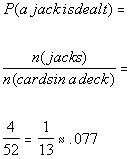*putting in the numbers found above *reducing the fraction  *rounding to 3 decimal places

 The probability that a jack is dealt is .077.Answer/Discussion to 2b Find the probability that you are dealt a heart or club.  Round answer to three decimal places.

Note that these two events are mutually exclusive.  A card CANNOT have a heart and a club on it at the same time.  So we can use the formula (property 4)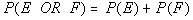Let's break it down first by finding each separate probability.

P(a heart is dealt)

 To find this probability we need to know n(E) = n(hearts), which is the number of hearts in a deck of cards and n(S) = n(cards in a deck), which is the total number of cards in a deck. What do you think n(E) = n(hearts) is?  If you said 13 you are correct.  There are 13 hearts in a deck of cards.   What do you think n(S) = n(cards in a deck) is?  If you said 52 give yourself a pat on the back.  There are 52 cards in a deck.   Putting this together in the Theoretical Probability formula you get: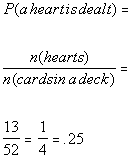*putting in the numbers found above *reducing the fraction  *rounding to 3 decimal places

P(a club is dealt)

 To find this probability we need to know n(E) = n(clubs), which is the number of clubs in a deck of cards and n(S) = n(cards in a deck), which is the total number of cards in a deck. What do you think n(E) = n(clubs) is?  If you said 13 you are correct.  There are 13 clubs in a deck of cards.   What do you think n(S) = n(cards in a deck) is?  If you said 52 give yourself a pat on the back.  There are 52 cards in a deck.   Putting this together in the Theoretical Probability formula you get: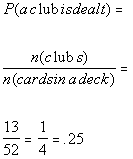*putting in the numbers found above *reducing the fraction  *rounding to 3 decimal places

Putting the two probabilities of mutually exclusive events together we get: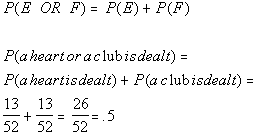*putting in the numbers found above *rounding to 3 decimal places

 The probability that a heart or club is dealt is .5.Answer/Discussion to 2c Find the probability that you are dealt a heart and a club.  Round answer to three decimal places.

 As mentioned in the Answer/Discussion to problem 2b, these two sets are mutually exclusive.  This time we are looking for the probability that is a heart AND a club is dealt.  This means the card must have both attributes.  Since they are mutually exclusive, this means they have nothing in common.  In other words, a card cannot be both a heart and a club at the same time.  So what would the probability of this event be???? If you said 0, you are right on.  Since n(a card with a heart and a club) is 0, the probability will be 0.Answer/Discussion to 2d Find the probability that you are dealt an ace and a spade.  Round answer to three decimal places.

 To find this probability we need to know n(E) = n(ace AND spade), which is the number of cards with an ace and a spade on it in a deck of cards and n(S) = n(cards in a deck), which is the total number of cards in a deck. What do you think n(E) = n(ace AND spade) is?  If you said 1 you are correct.  There is 1 card in a deck of cards that has both an ace and a spade on it.   What do you think n(S) = n(cards in a deck) is?  If you said 52 give yourself a pat on the back.  There are 52 cards in a deck.   Putting this together in the Theoretical Probability formula you get: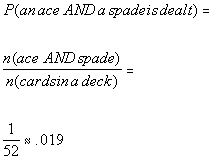*putting in the numbers found above *rounding to 3 decimal places

 The probability that a card with an ace and spade on it is dealt is .019.Answer/Discussion to 2e Find the probability that you are dealt an ace or a spade.  Round answer to three decimal places.

Note that these two events are NOT mutually exclusive.  A card CAN have an ace or a spade at the same time.  So we can use the formula (property 5)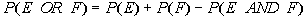Let's break it down first by finding each separate probability.

P(an ace is dealt)

 To find this probability we need to know n(E) = n(aces), which is the number of aces in a deck of cards and n(S) = n(cards in a deck), which is the total number of cards in a deck. What do you think n(E) = n(aces) is?  If you said 4 you are correct.  There are 4 aces in a deck of cards.   What do you think n(S) = n(cards in a deck) is?  If you said 52 give yourself a pat on the back.  There are 52 cards in a deck.   Putting this together in the Theoretical Probability formula you get: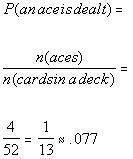*putting in the numbers found above *reducing the fraction  *rounding to 3 decimal places

P(a spade is dealt)

 To find this probability we need to know n(E) = n(spades), which is the number of spades in a deck of cards and n(S) = n(cards in a deck), which is the total number of cards in a deck. What do you think n(E) = n(spades) is?  If you said 13 you are correct.  There are 13 spades in a deck of cards.   What do you think n(S) = n(cards in a deck) is?  If you said 52 give yourself a pat on the back.  There are 52 cards in a deck.   Putting this together in the Theoretical Probability formula you get: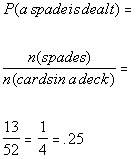*putting in the numbers found above *reducing the fraction  *rounding to 3 decimal places

 P(an ace AND a spade is dealt) We found this probability to be 1/52 in the Answer/Discussion to problem 2d.  Putting the probabilities of non mutually exclusive events together we get: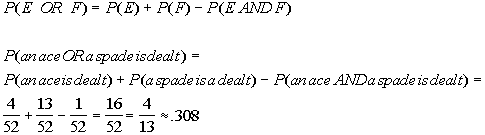*putting in the numbers found above *rounding to 3 decimal places

 The probability that a card that is dealt has an ace or a spade on it is .308.Answer/Discussion to 3a Find the probability that 2 freshmen, 2 sophomores and 2 juniors are selected.  Round answer to three decimal places.

 To find this probability we need to know n(E) = n(2 freshmen, 2 sophomores and 2 juniors are selected), which is the number of ways 6 names drawn can contain 2 freshmen, 2 sophomores and 2 juniors and n(S) = n(6 name drawings), which is the total number of ways 6 names can be drawn. What do you think n(E) = n(2 freshmen, 2 sophomores and 2 juniors are selected) is?  Since we are counting draws that means we are counting groups of names, which means we need to use combinations to count them.  If you need a review on combinations, feel free to go to Tutorial 57: Combinations. If you said 21168 you are correct.  Let's see how we get that number. Here is what the committee looks like:  we would have to have 2 freshmen, 2 sophomores and 2 juniors to meet this condition:  2 FRESHMEN    2 SOPHOMORES   2 JUNIORS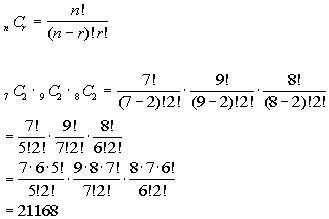*Comb. of n = 7 freshmen taken r = 2 at a time *Comb. of n = 9 sophomores taken r = 2 at a time *Comb. of n = 8 juniors taken r = 2 at a time   *Expand 7! until it gets to 5!, 9! until it gets to 7!, and 8! until it gets to 6!  which are the larger !'s in the den. *Cancel out 5!'s, 7!'s, and 6!'s

 As mentioned above, n(E) = n(2 freshmen, 2 sophomores and 2 juniors are selected) = 21168. If you have a factorial key, you can put it in as 7!, divided by 5!, divided by 2! times 9!, divided by 7!, divided by 2!, times 8!, divided by 6!, divided by 2! and then press enter or =.  If you don't have a factorial key, you can simplify it as shown above and then enter it in.

 What do you think n(S) = n(6 name drawings) is?  If you said 1344904 give yourself a pat on the back.  Again you would have to use combinations to figure this one out.  Overall, there are 7 freshmen, 9 sophomores, 8 juniors, and 10 seniors for a total of 34 students, taken 6 at a time.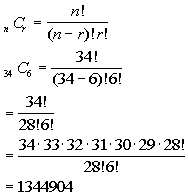*Comb. of n = 34 students taken r = 6 at a time *Eval. inside ( ) *Expand 34! until it gets to 28!   which is the larger ! in the den. *Cancel out 26!'s

 As mentioned above, n(S) = n(6 name drawings) = 1344904. If you have a factorial key, you can put it in as 34!, divided by 28!, divided by 6! and then press enter or =.  If you don't have a factorial key, you can simplify it as shown above and then enter it in.    Putting this together in the Theoretical Probability formula you get: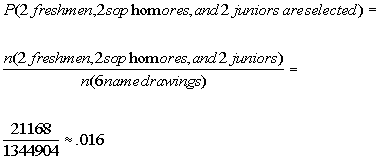*putting in the numbers found above *rounding to 3 decimal places

 The probability that 2 freshmen, 2 sophomores and 2 juniors are drawn is .016.Answer/Discussion to 3b Find the probability that only seniors are selected.  Round answer to three decimal places.

 To find this probability we need to know n(E) = n(only seniors are drawn), which is the number of ways 6 names drawn can contain only seniors and n(S) = n(6 name drawings), which is the total number of ways 6 names can be drawn. What do you think n(E) = n(seniors) is?  Since we are counting draws that means we are counting groups of names, which means we need to use combinations to count them.  If you need a review on combinations, feel free to go to Tutorial 57: Combinations. If you said 210 you are correct.  Let's see how we get that number. Here is what the committee looks like:  we would have to have only seniors to meet this condition:  6 SENIORS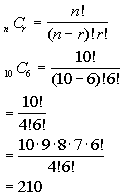*Comb. of n = 10 seniors taken r = 6 at a time *Eval. inside ( ) *Expand 10! until it gets to 6! which is the larger ! in the den. *Cancel out 6!'s

 As mentioned above, n(E) = n(only seniors are drawn) = 210. If you have a factorial key, you can put it in as 10!, divided by 4!, divided by 6! and then press enter or =.  If you don't have a factorial key, you can simplify it as shown above and then enter it in.

 What do you think n(S) = n(6 name drawings) is?  As shown in Answer/Discussion to problem 3a, n(S) = n(6 name drawings) = 1344904.   Putting this together in the Theoretical Probability formula you get: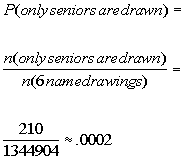*putting in the numbers found above *rounding to 4 decimal places

 The probability that only seniors are drawn is .0002. Note I carried this out 4 decimal places since that was the first non zero digit.Answer/Discussion to 4a Find the probability that a six is rolled the 1st time and an odd number is rolled a 2nd time.  Round answer to three decimal places.

 Since the outcome of the 1st roll of the die does NOT affect the outcome of the 2nd roll of the die, this means these two events are independent.  So we can use the formulato find our probability. Let's break it down.

P(1st roll is a 6)

 To find this probability we need to know n(E) = n(6's on the die), which is the number of 6's on a die and n(S) = n(numbers on a die), which is the total number of numbers on a die. What do you think n(E) = n(6's on the die) is?  If you said 1 you are correct. There is 1 number 6 on a die.   What do you think n(S) = n(numbers on a die) is?  If you said 6 give yourself a pat on the back. There are 6 numbers on a die. Putting this together in the Theoretical Probability formula you get: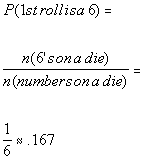*putting in the numbers found above *rounding to 3 decimal places

P(2nd roll is odd)

 To find this probability we need to know n(E) = n(odd numbers on a die), which is the number of odd numbers on a die and n(S) = n(numbers on a die), which is the total number of numbers on a die. What do you think n(E) = n(odd numbers on a die) is?  If you said 3 you are correct. There is 3 odd numbers on a die.   What do you think n(S) = n(numbers on a die) is?  If you said 6 give yourself a pat on the back. There are 6 numbers on a die. Putting this together in the Theoretical Probability formula you get: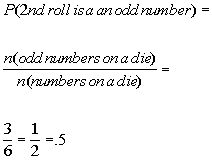*putting in the numbers found above *rounding to 3 decimal places

Putting the probabilities of two events that are independent together we get: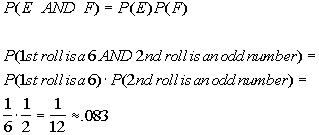*putting in the numbers found above *rounding to 3 decimal placesAnswer/Discussion to 4b Find the probability that a number less than or equal to 2 is rolled the 1st time and an even number is rolled the 2nd time.  Round answer to three decimal places.

 Since the outcome of the 1st roll of the die does NOT affect the outcome of the 2nd roll of the die, this means these two events are independent.  So we can use the formulato find our probability. Let's break it down.

P(1st roll is less than or equal to 2)

 To find this probability we need to know n(E) = n(numbers less than or equal to 2), which is the number of numbers that are less than or equal to 2 on a die and n(S) = n(numbers on a die), which is the total number of numbers on a die. What do you think n(E) = n(numbers less than or equal to 2) is?  If you said 2 you are correct. There are 2 numbers that are less than or equal to 2 on a die.   What do you think n(S) = n(numbers on a die) is?  If you said 6 give yourself a pat on the back. There are 6 numbers on a die. Putting this together in the Theoretical Probability formula you get: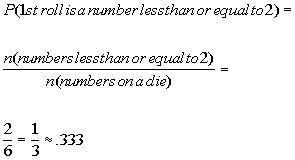*putting in the numbers found above *rounding to 3 decimal places

P(2nd roll is even)

 To find this probability we need to know n(E) = n(even numbers on a die), which is the number of even numbers on a die and n(S) = n(numbers on a die), which is the total number of numbers on a die. What do you think n(E) = n(even numbers on a die) is?  If you said 3 you are correct. There is 3 even numbers on a die.   What do you think n(S) = n(numbers on a die) is?  If you said 6 give yourself a pat on the back. There are 6 numbers on a die. Putting this together in the Theoretical Probability formula you get: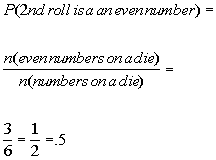*putting in the numbers found above *rounding to 3 decimal places

Putting the probabilities of two events that are independent together we get: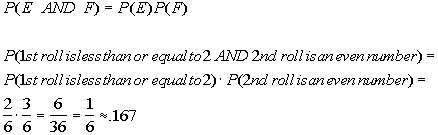*putting in the numbers found above *rounding to 3 decimal places

Last revised on May 16, 2011 by Kim Seward.
All contents copyright (C) 2002 - 2011, WTAMU and Kim Seward. All rights reserved.